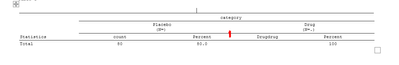## Proc report Spanning line break between categories header

I want to have a break in the spanning line between the categories ( Indicated n the Image in Red).  Is it possible to do that? Thank you for your suggestions.``````data check;
pla = '80';
drug= '100';
col1=' Total';
pla_pct= '80.0';
drug_pct = '100';
run;

ods _all_ close;
options orientation = landscape errors = 2 missing = ' ' nobyline;
ods escapechar = '^';
Ods results on;
ods rtf file = "c:temp\check.rtf" style = custom;
proc report data=check  ls = 145 ps = 55  split = "?" headline center missing formchar(2) = '_'
;

title1 j = l "Table 1";

column   ("^R/RTF'\brdrb\brdrs '" col1
("(*ESC*)S={borderbottomcolor=black borderbottomwidth=2}	category"
("(*ESC*)S={borderbottomcolor=black borderbottomwidth=2} Placebo ?(N=)"
pla pla_pct )
("(*ESC*)S={borderbottomcolor=black borderbottomwidth=2} Drug ?(N=.)"
Drugdrug drug_pct)));

define col1  	/ 'Statistics' order
define pla	    /  "count"
style(header) = [just=c] style(column) = [just=c vjust=b  	cellwidth=11% asis=on] ;
define pla_pct	    /  "Percent"
style(header) = [just=c] style(column) = [just=c vjust=b  	cellwidth=11% asis=on];
define drug	    / "count"
style(header) = [just=c] style(column) = [just=c vjust=b  	cellwidth=11% asis=on] ;
define drug_pct	    / "Percent"
style(header) = [just=c] style(column) = [just=c vjust=b 	cellwidth=11% asis=on];

run;
ods rtf close;
ods listing close;

``````

1 ACCEPTED SOLUTION

Accepted SolutionsKsharp
Super User

## Re: Proc report Spanning line break between categories header

``````data check;
pla = '80';
drug= '100';
col1=' Total';
pla_pct= '80.0';
drug_pct = '100';
run;

options orientation = landscape errors = 2 missing = ' ' nobyline;
ods escapechar = '^';
ods rtf file = "c:\temp\check.rtf" style = journal;
proc report data=check  ls = 145 ps = 55  split = "?" nowd headline center missing formchar(2) = '_'
;

title1 j = l "Table 1";

column   ("^R/RTF'\brdrb\brdrs '" col1
("(*ESC*)S={borderbottomcolor=black borderbottomwidth=2}	category"
("(*ESC*)S={borderbottomcolor=black borderbottomwidth=2} Placebo ?(N=)"
pla pla_pct )
dummy
("(*ESC*)S={borderbottomcolor=black borderbottomwidth=2} Drug ?(N=.)"
drug drug_pct)));

define col1  	/ 'Statistics' order
define pla	    /  "count"
style(header) = [just=c] style(column) = [just=c vjust=b  	cellwidth=11% asis=on] ;
define pla_pct	    /  "Percent"
style(header) = [just=c] style(column) = [just=c vjust=b  	cellwidth=11% asis=on];
define drug	    / "count"
style(header) = [just=c] style(column) = [just=c vjust=b  	cellwidth=11% asis=on] ;
define drug_pct	    / "Percent"
style(header) = [just=c] style(column) = [just=c vjust=b 	cellwidth=11% asis=on];
define dummy/computed ' ';
compute dummy/character length=1;
dummy=' ';
endcomp;
run;
ods rtf close;
``````4 REPLIES 4

## Re: Proc report Spanning line break between categories header

Since you are using a CUSTOM style in your ODS RTF output you should share the code for that style as well.

It isn't impossible for us to come up with a solution that may work for some ODS Styles but not the one you are using as we do not have your style.

## Re: Proc report Spanning line break between categories header

Thanks @ballardw  for your response. Unfortunately, I couldn't find the code where it is stored in my organization. They are set up in such a way it reads into the SAS automatically.Ksharp
Super User

## Re: Proc report Spanning line break between categories header

``````data check;
pla = '80';
drug= '100';
col1=' Total';
pla_pct= '80.0';
drug_pct = '100';
run;

options orientation = landscape errors = 2 missing = ' ' nobyline;
ods escapechar = '^';
ods rtf file = "c:\temp\check.rtf" style = journal;
proc report data=check  ls = 145 ps = 55  split = "?" nowd headline center missing formchar(2) = '_'
;

title1 j = l "Table 1";

column   ("^R/RTF'\brdrb\brdrs '" col1
("(*ESC*)S={borderbottomcolor=black borderbottomwidth=2}	category"
("(*ESC*)S={borderbottomcolor=black borderbottomwidth=2} Placebo ?(N=)"
pla pla_pct )
dummy
("(*ESC*)S={borderbottomcolor=black borderbottomwidth=2} Drug ?(N=.)"
drug drug_pct)));

define col1  	/ 'Statistics' order
define pla	    /  "count"
style(header) = [just=c] style(column) = [just=c vjust=b  	cellwidth=11% asis=on] ;
define pla_pct	    /  "Percent"
style(header) = [just=c] style(column) = [just=c vjust=b  	cellwidth=11% asis=on];
define drug	    / "count"
style(header) = [just=c] style(column) = [just=c vjust=b  	cellwidth=11% asis=on] ;
define drug_pct	    / "Percent"
style(header) = [just=c] style(column) = [just=c vjust=b 	cellwidth=11% asis=on];
define dummy/computed ' ';
compute dummy/character length=1;
dummy=' ';
endcomp;
run;
ods rtf close;
``````## Re: Proc report Spanning line break between categories header

Thank you @Ksharp. You Rocks...........

Discussion stats
• 4 replies
• 247 views
• 1 like
• 3 in conversation